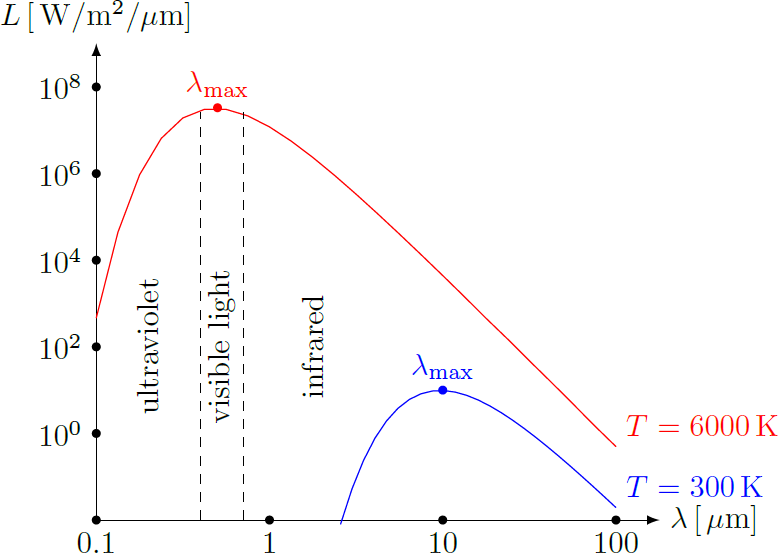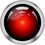# Atmospheric physics 11: Thermal radiationDue to their temperature, all bodies emit thermal radiation, which is electromagnetic radiation. This radiation is also often called black-body radiation, since the theory assumes a body, that is completely opaque and non-reflective. Depending on the temperature of the body, microwaves, infrared radiation, visible light, UV radiation or even X-ray radiation can be emitted to certain proportions. The maximum radiation occurs for a black body at a wavelength $\lambda_\text{max}$, which results from Wien's law: $\boxed{\lambda_\text{max} \approx \dfrac{2898 \,\text{µm}\,\text{K}}{T} }$ where $T$ denotes the abosolute temperature in Kelvin. The complete spectrum of thermal radiation resembles a bell curve with a maximum at $\lambda_\text{max}$, as shown in the diagram to the right (Planck's law). With increasing temperature, the maximum shifts to smaller wavelengths and higher frequencies. While the sun, with a surface temperature of around 6000 Kelvin, emits a major part of the radiation as visible light ($\lambda_\text{max} \approx 500 \,\text{nm}$), the radiation peaks at room temperature in the mid-infrared range ($\lambda_\text{max} \approx 10 \,\text{µm}$).

But not only the wavelength range, but also the total power of the heat radiation (area under the spectrum curve) depends on the temperature of the body. The total intensity of thermal radiation (power per surface area) follows the Stefan–Boltzmann law: $\boxed{ I = \dfrac{P}{A} = \varepsilon \cdot \sigma \cdot T^4 }$ where $\sigma = 5.67 \cdot 10^{-8} \,\text{W}/\text{m}^2/\text{K}^2$ is the Stefan-Boltzmann constant and $0 < \varepsilon \leq 1$ is the so-called emissivity. Emissivity represents the effectiveness with which a body releases thermal radiation. An ideal black body corresponds to the case $\varepsilon = 1$, while a body, that does not absorb all incident radiation (a gray body), is characterized by an emissivity $\varepsilon < 1$. Note, that due to the fourth power in the Stefan-Boltzmann law, a doubling of the temperature means a 16-fold increase in thermal radiation.Note by Markus Michelmann
3 years, 3 months ago

This discussion board is a place to discuss our Daily Challenges and the math and science related to those challenges. Explanations are more than just a solution — they should explain the steps and thinking strategies that you used to obtain the solution. Comments should further the discussion of math and science.

When posting on Brilliant:

• Use the emojis to react to an explanation, whether you're congratulating a job well done , or just really confused .
• Ask specific questions about the challenge or the steps in somebody's explanation. Well-posed questions can add a lot to the discussion, but posting "I don't understand!" doesn't help anyone.
• Try to contribute something new to the discussion, whether it is an extension, generalization or other idea related to the challenge.
• Stay on topic — we're all here to learn more about math and science, not to hear about your favorite get-rich-quick scheme or current world events.

MarkdownAppears as
*italics* or _italics_ italics
**bold** or __bold__ bold
- bulleted- list
• bulleted
• list
1. numbered2. list
1. numbered
2. list
Note: you must add a full line of space before and after lists for them to show up correctly
paragraph 1paragraph 2

paragraph 1

paragraph 2

[example link](https://brilliant.org)example link
> This is a quote
This is a quote
    # I indented these lines
# 4 spaces, and now they show
# up as a code block.

print "hello world"
# I indented these lines
# 4 spaces, and now they show
# up as a code block.

print "hello world"
MathAppears as
Remember to wrap math in $$ ... $$ or $ ... $ to ensure proper formatting.
2 \times 3 $2 \times 3$
2^{34} $2^{34}$
a_{i-1} $a_{i-1}$
\frac{2}{3} $\frac{2}{3}$
\sqrt{2} $\sqrt{2}$
\sum_{i=1}^3 $\sum_{i=1}^3$
\sin \theta $\sin \theta$
\boxed{123} $\boxed{123}$

## Comments

There are no comments in this discussion.

×

Problem Loading...

Note Loading...

Set Loading...